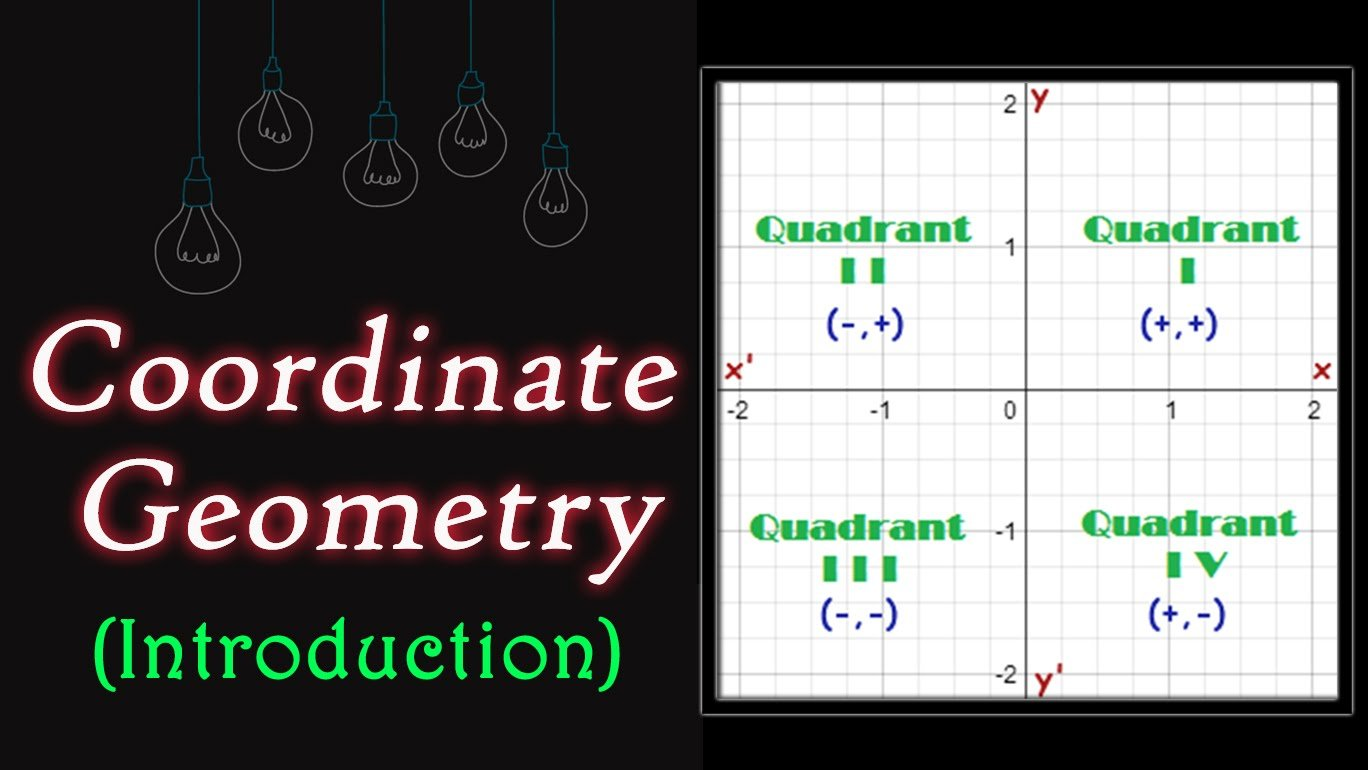Coordinate geometry, also known as analytic geometry or Cartesian geometry, is a branch of classical mathematics to study geometry using a coordinate system. Here, the positions of points on the plane is described using ordered pairs of numbers. The coordinate plane uses two scales: x-axis and y-axis. The system was initially proposed by Rene Descartes.

The chapter of coordinate geometry in Class 10 mathematics covers following topics:
• Introduction
• Distance Formula
• Section Formula
• Area of a Triangle
• Summary

The NCERT solutions for class 10th maths chapter 7 Coordinate geometry includes the topics to find the distance between two points using coordinates, and to find the coordinates of the point which divides a line segment in a given ratio. In this chapter, the method to find the area of the triangle formed by three given points is also discussed.

Distance Formula: This topic mainly highlights the bunch of formulas used in coordinate geometry to find the distance between two given points denoted by their respective coordinates. Distance in one-dimension, two-dimensions and three-dimensions consider different formulas. In case of two- dimensions, two points lying on an axis, say x-axis is obtained by calculating the absolute value of the difference of their x-coordinates. Midpoint Formula and Distance Formula are commonly used to find out the distance between two points with their coordinates given.

Section Formula: The section formula in coordinate geometry gives the co-ordinates of the point which divides the given line segment into two in a given ratio (m:n). It is widely used to find out incenter, centroid and excenters of a triangle. In physics, it is used to find out the center of mass, equilibrium points, etc. Internal divisions and External divisions can be found out with the help of section formula.

Area of Triangle: A triangle is a polygon with three edges and three vertices. This section discusses how to find area of a triangle with the coordinates of its vertices given. Given the coordinates of the three vertices A,B and C, the area of triangle is given by:

Area= Ax (By-Cy)+ Bx (Cy- Ay)+ Cx (Ay- By)/2

, where x and y are the coordinates of respective vertices.

The formula can be used even when the coordinates are negative also. If the value obtained with the formula is ‘Zero’, then it means that three points are collinear. Alternatively, by finding the length of each sides using Distance formula, the area of a given triangle can be calculated using Heron’s formula.

With these topics being discussed in CBSE class 10 maths coordinate geometry, it is understood that with coordinates given, it is possible to:
• Find the midpoint, slope and equation of a line segment.
• Determine the distance between two given points.
• Determine the perpendicularity and parallelism of lines.
• Form or transform shapes by changing the location of points.
• Define equations based on coordinates for curves, circles, and ellipses
• Find the area and perimeter of polygons defined by the given points.